Miscellaneous

Chapter 3 Class 12 Matrices
Serial order wise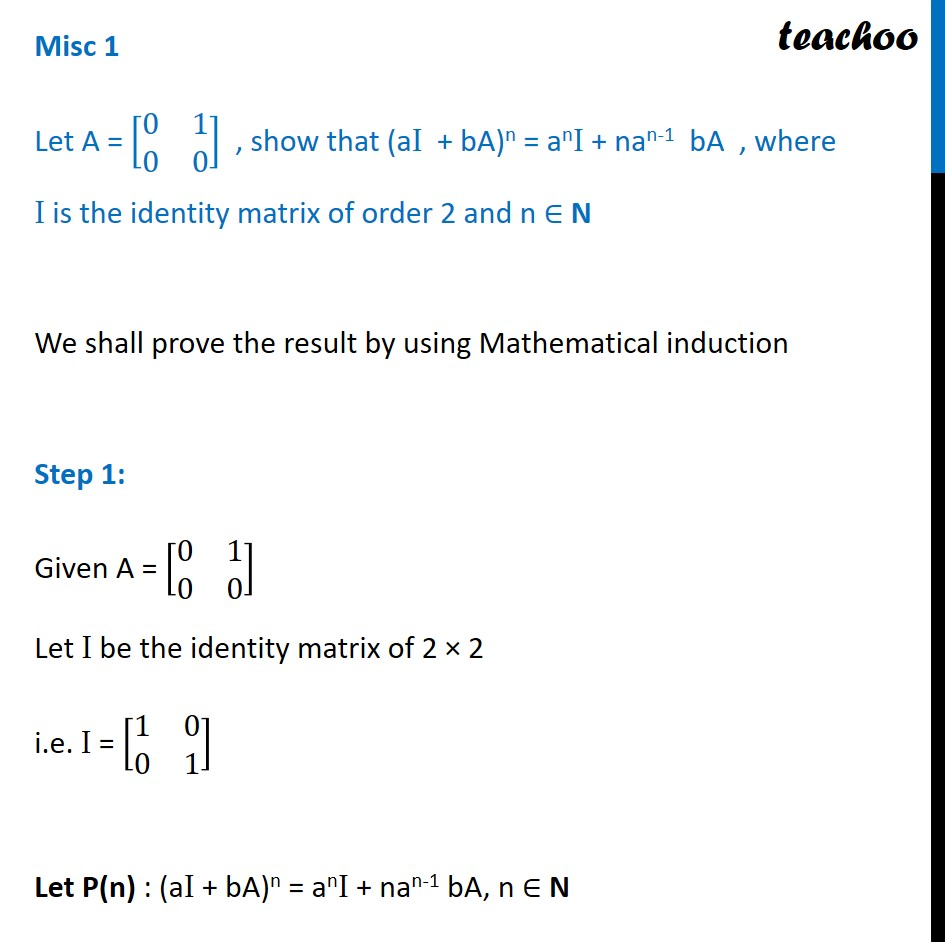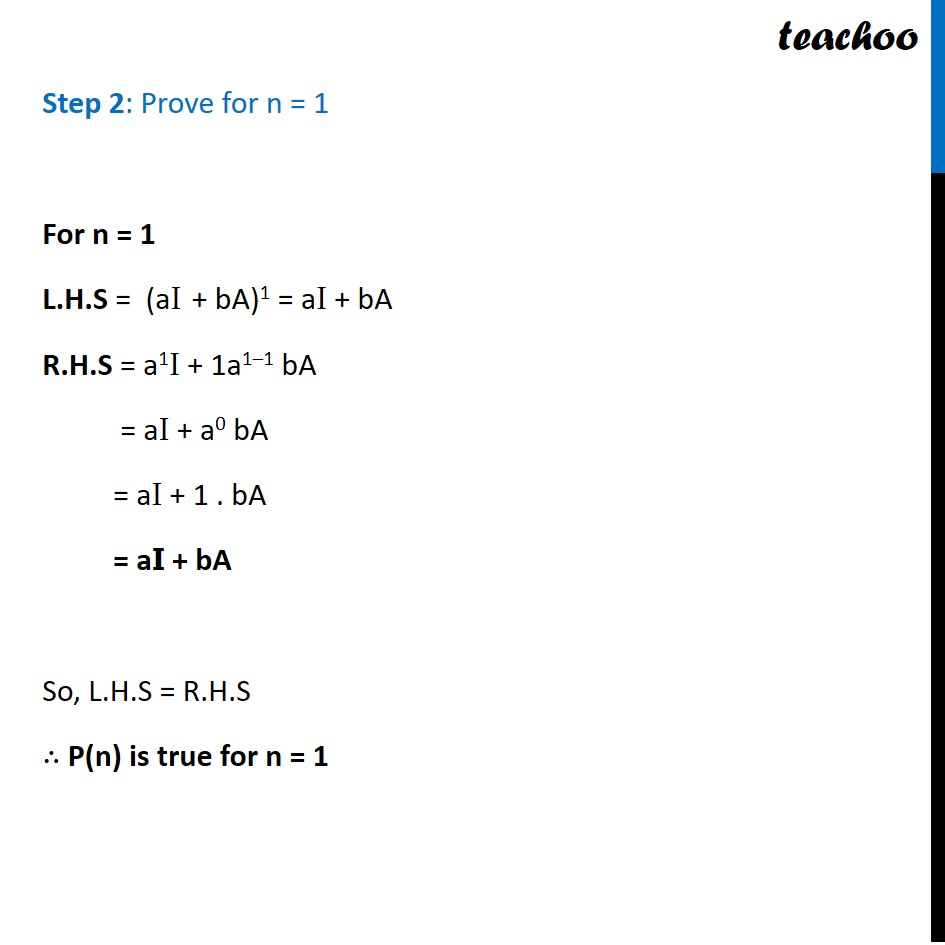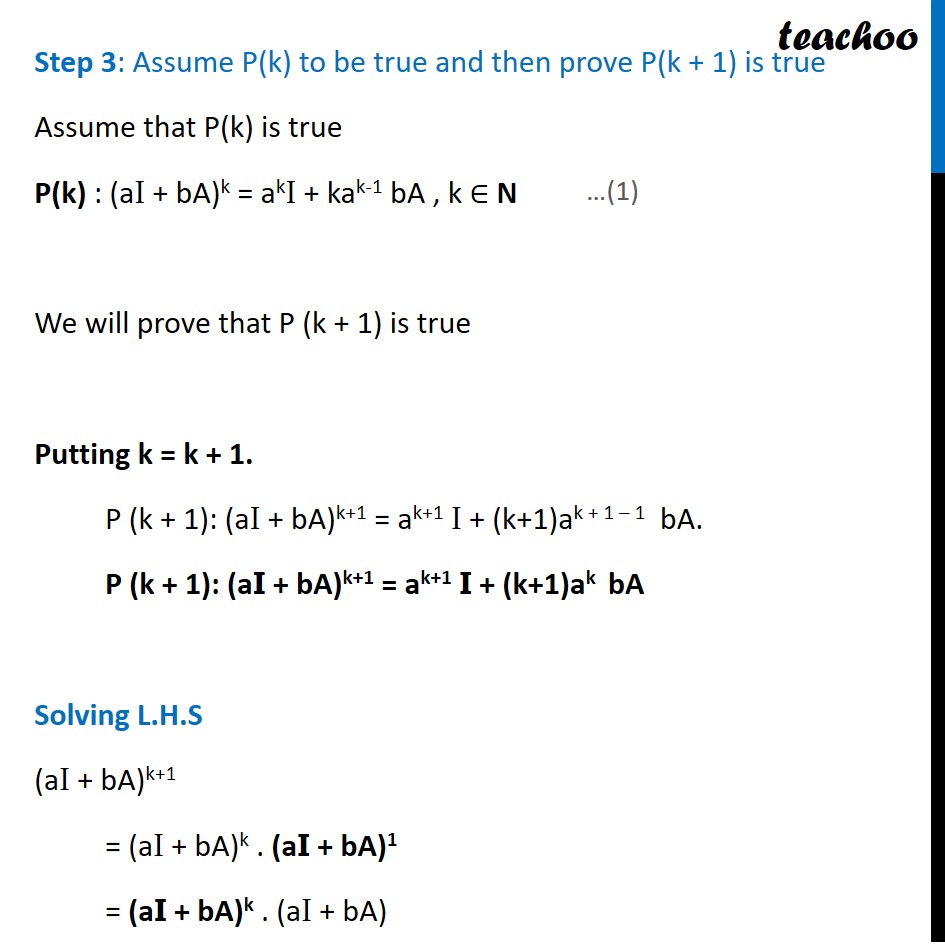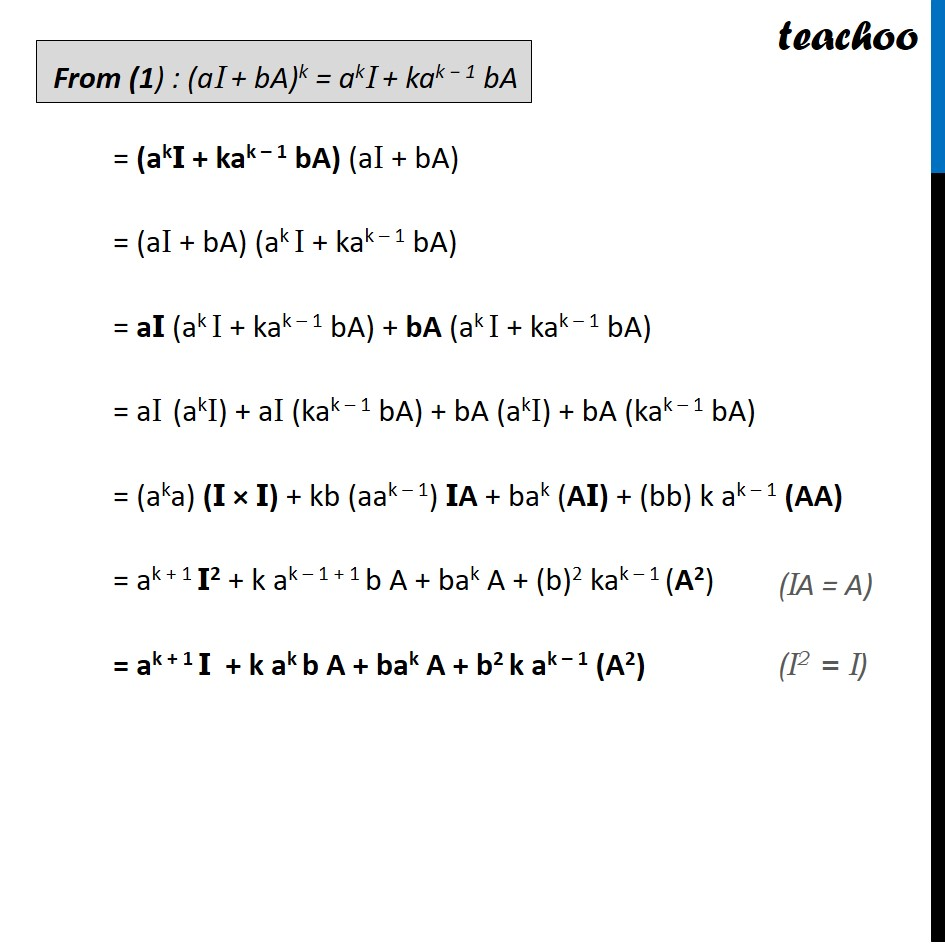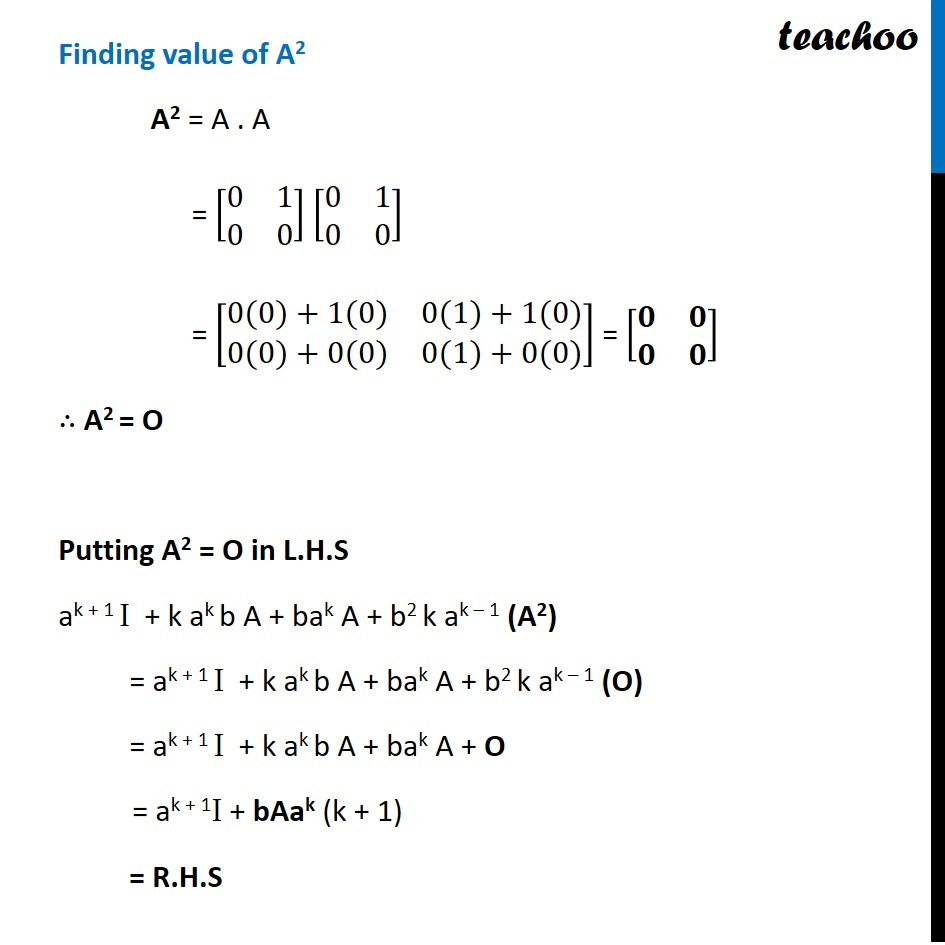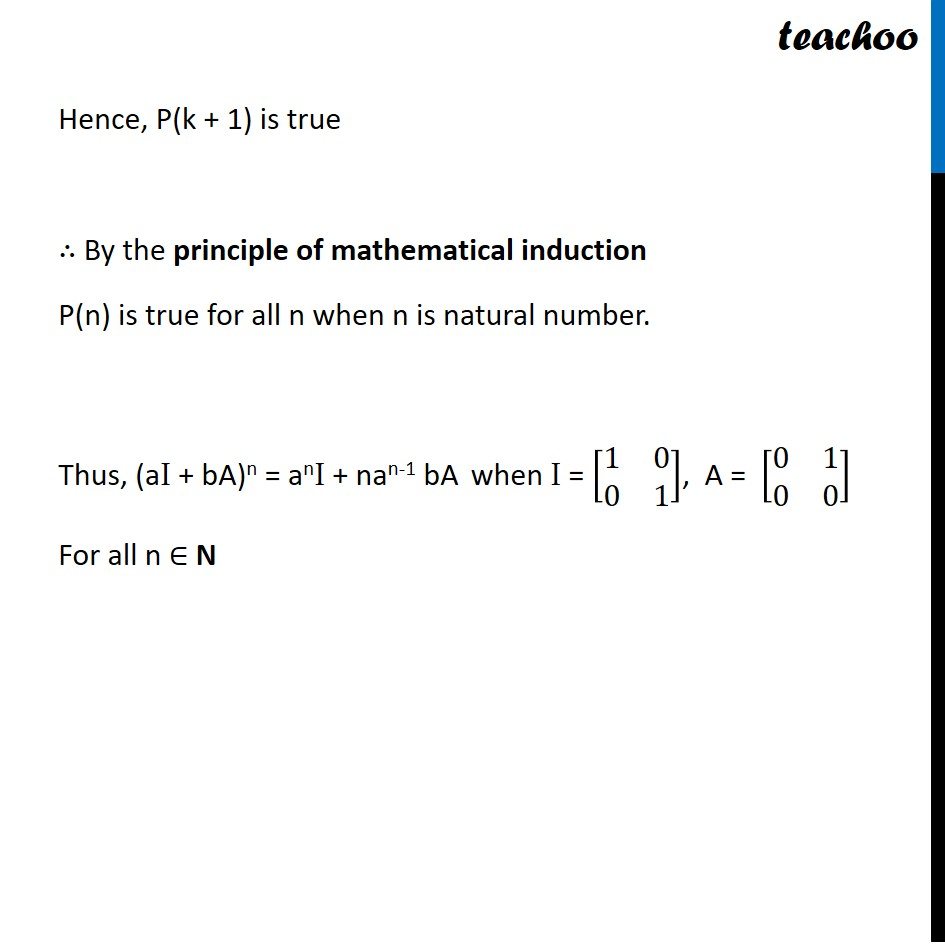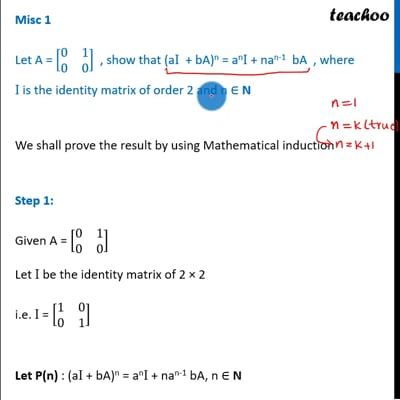This video is only available for Teachoo black users

Solve all your doubts with Teachoo Black (new monthly pack available now!)

### Transcript

Misc 1 Let A = [■8(0&1@0&0)] , show that (aI + bA)n = anI + nan-1 bA , where I is the identity matrix of order 2 and n ∈ N We shall prove the result by using Mathematical induction Step 1: Given A = [■8(0&1@0&0)] Let I be the identity matrix of 2 × 2 i.e. I = [■8(1&0@0&1)] Let P(n) : (aI + bA)n = anI + nan-1 bA, n ∈ N Step 2: Prove for n = 1 For n = 1 L.H.S = (aI + bA)1 = aI + bA R.H.S = a1I + 1a1–1 bA = aI + a0 bA = aI + 1 . bA = aI + bA So, L.H.S = R.H.S ∴ P(n) is true for n = 1 Step 3: Assume P(k) to be true and then prove P(k + 1) is true Assume that P(k) is true P(k) : (aI + bA)k = akI + kak-1 bA , k ∈ N We will prove that P (k + 1) is true Putting k = k + 1. P (k + 1): (aI + bA)k+1 = ak+1 I + (k+1)ak + 1 – 1 bA. P (k + 1): (aI + bA)k+1 = ak+1 I + (k+1)ak bA Solving L.H.S (aI + bA)k+1 = (aI + bA)k . (aI + bA)1 = (aI + bA)k . (aI + bA) From (1) : (aI + bA)k = akI + kak − 1 bA = (akI + kak – 1 bA) (aI + bA) = (aI + bA) (ak I + kak – 1 bA) = aI (ak I + kak – 1 bA) + bA (ak I + kak – 1 bA) = aI (akI) + aI (kak – 1 bA) + bA (akI) + bA (kak – 1 bA) = (aka) (I × I) + kb (aak – 1) IA + bak (AI) + (bb) k ak – 1 (AA) = ak + 1 I2 + k ak – 1 + 1 b A + bak A + (b)2 kak – 1 (A2) = ak + 1 I + k ak b A + bak A + b2 k ak – 1 (A2) Finding value of A2 A2 = A . A = [■8(0&1@0&0)] [■8(0&1@0&0)] = [■8(0(0)+1(0)&0(1)+1(0)@0(0)+0(0)&0(1)+0(0))] = [■8(𝟎&𝟎@𝟎&𝟎)] ∴ A2 = O Putting A2 = O in L.H.S ak + 1 I + k ak b A + bak A + b2 k ak – 1 (A2) = ak + 1 I + k ak b A + bak A + b2 k ak – 1 (O) = ak + 1 I + k ak b A + bak A + O = ak + 1I + bAak (k + 1) = R.H.S Hence P(k + 1) is true Hence, P(k + 1) is true ∴ By the principle of mathematical induction P(n) is true for all n when n is natural number. Thus, (aI + bA)n = anI + nan-1 bA when I = [■8(1&0@0&1)], A = [■8(0&1@0&0)] For all n ∈ N